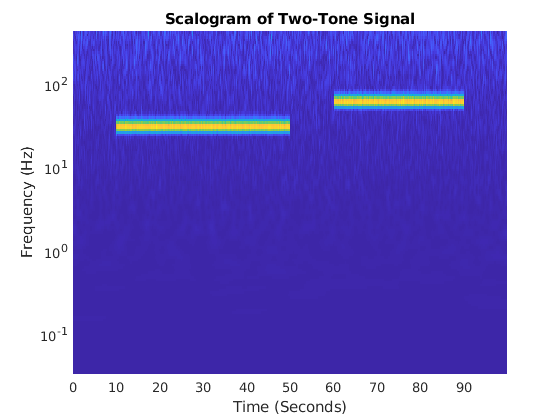# CUDA Code from CWT

This example shows how to generate a MEX file to perform the continuous wavelet transform (CWT) using generated CUDA code.

First, ensure that you have a CUDA-enabled GPU and the NVCC compiler. See The GPU Environment Check and Setup App (GPU Coder) to ensure you have the proper configuration.

Create a GPU coder configuration object.

`cfg = coder.gpuConfig("mex");`

Generate a signal of 100,000 samples at 1,000 Hz. The signal consists of two cosine waves with disjoint time supports.

```t = 0:.001:(1e5*0.001)-0.001; x = cos(2*pi*32*t).*(t > 10 & t<=50)+ ... cos(2*pi*64*t).*(t >= 60 & t < 90)+ ... 0.2*randn(size(t));```

Cast the signal to use single precision. GPU calculations are often more efficiently done in single precision. You can however also generate code for double precision if your NVIDIA GPU supports it.

`x = single(x);`

Generate the GPU MEX file and a code generation report. To allow generation of the MEX file, you must specify the properties (class, size, and complexity) of the three input parameters:

• `coder.typeof(single(0),[1 1e5])` specifies a row vector of length 100,000 containing real `single` values.

• `coder.typeof('c',[1 inf])` specifies a character array of arbitrary length.

• `coder.typeof(0)` specifies a real `double` value.

```sig = coder.typeof(single(0),[1 1e5]); wav = coder.typeof('c',[1 inf]); sfrq = coder.typeof(0); codegen cwt -config cfg -args {sig,wav,sfrq} -report```
```Code generation successful: View report ```

The -report flag is optional. Using `-report` generates a code generation report. In the Summary tab of the report, you can find a GPU code metrics link, which provides detailed information such as the number of CUDA kernels generated and how much memory was allocated.

Run the MEX file on the data and plot the scalogram. Confirm the plot is consistent with the two disjoint cosine waves.

```[cfs,f] = cwt_mex(x,'morse',1e3); image("XData",t,"YData",f,"CData",abs(cfs),"CDataMapping","scaled") set(gca,"YScale","log") axis tight xlabel("Time (Seconds)") ylabel("Frequency (Hz)") title("Scalogram of Two-Tone Signal")```Run the CWT command above without appending the `_``mex`. Confirm the MATLAB and the GPU MEX scalograms are identical.

```[cfs2,f2] = cwt(x,'morse',1e3); max(abs(cfs2(:)-cfs(:)))```
```ans = single 7.3380e-07 ```